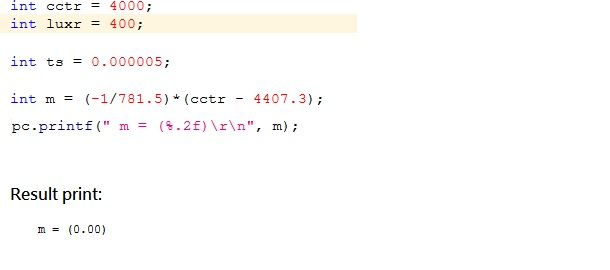## How to calculate and display the digit after the decimal point?

I only remember that I can use printf(%.f) to display the digit after the decimal point, but I do not know that how to calculate and result the digit after the decimal point (only can display 0.00 like image photo). What the code I need for calculate? Please give me the code or example page, thank you.##### 3 years, 3 months ago.

you declared 'm' to be an int, so any fractional value will ultimately be truncated. Try changing

```float m = (-1/781.5)*(cctr-4407.3);
```

There may be other sources of truncation in this formula that you might explore. Change the "-1" to "-1.0", and the "cctr" to "(float)cctr" - if necessary. At least from the human reviewer perspective, the intent is then more clear even if the compiler does the right thing.